# Hessian of a function

Jump to: navigation, searchThe quadratic form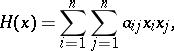or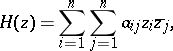where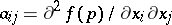(or) andis given on the-dimensional real space(or on the complex space) with coordinates(or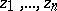). Introduced in 1844 by O. Hesse. With the aid of a local coordinate system this definition is transferred to functions defined on a real manifold of class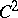(or on a complex space), at critical points of the functions. In both cases the Hessian is a quadratic form given on the tangent space and is independent of the choice of coordinates. In Morse theory the Hessian is used to define the concepts of a (non-)degenerate critical point, the Morse form and the Bott form. In complex analysis the Hessian is used in the definition of a pseudo-convex space (cf. Pseudo-convex and pseudo-concave) and of a plurisubharmonic function.